## How to use the group produced with DirectProduct?...

I don't know how to use the group produced with `DirectProduct`. such as

```G := DirectProduct(QuaternionGroup(), CyclicGroup(3)):
DrawSubgroupLattice(G)```

Or

`IsNormal(CyclicGroup(2), DirectProduct(CyclicGroup(2), CyclicGroup(2)))`

They all will get error information. It looks like the format is different, do I need to convert it somehow? Or is this a bug in maple?

## coloring surfaces in a plot...

Hello,

Im a tax student and tring to figure out Maple. I plotted the following graph with the code:

implicitplot([Vth = Vps, V1 = 25000, V1 = 50000, V1 = 75000, V1 = 100000], E = 0 .. 1000000, T = 0 .. 15, color = [black], labels = ["E in (EUR)", "T (in Jahren)"], labelfont = [times, bold, 12], view = [0 .. 1000000, 0 .. 15])I would like to color the intermediate areas and attach a legend. A colleague has plotted this for me, but unfortunately has not provided me with the source code and has now lost the file. Can someone help me to re-plot the last shown chart?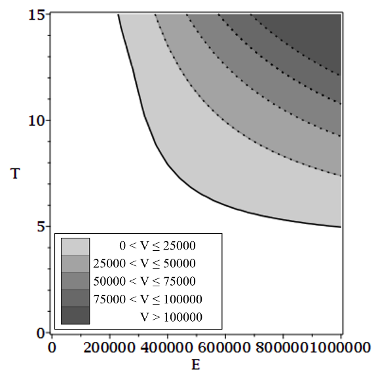Thanks so so much in advance!! Best regards Rebekka

## Draw indifference curve in 3d plot...

Hello,

Im a tax student trying to use Maple for my studies. I plotted two surfaces in a 3D coordinate system, the surfaces intersect = indifference area.

The current formula is like this:

plot3d([Vth/E, Vps/E], E = 0 .. 1000000, T = 0 .. 15, labels = ["E", "T", ""], color = [gray, white], labels = ["E (in EUR)", "T (in Jahren)", ""], labelfont = [times, bold, 12])
It looks like this: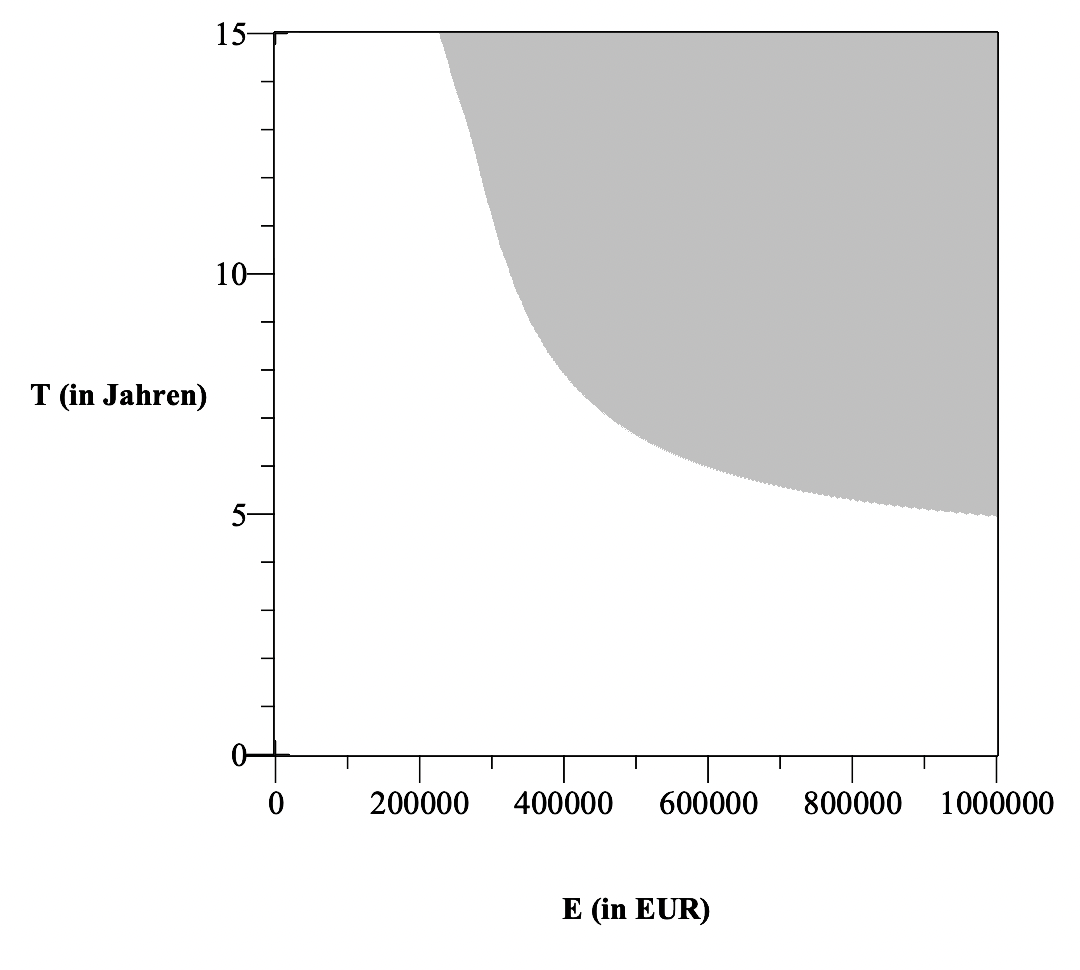I would like to draw in the same graph, the indifference area (Vth/E = Vth/E), it looks like this: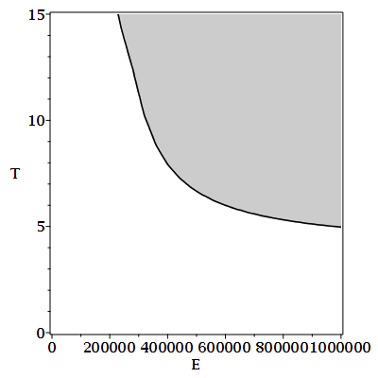A colleague has plotted this for me, but unfortunately has not provided me with the source code and has now lost the file. Can someone help me to re-plot the last shown chart?

Rebekka

## Why does the "D" operator behave different in the ...

I want to explore multivariable function approximations using truncated Taylor series.

Mathematically, for a function f(x,y) and using operator notation for the partial derivatives, where e.g. Dx2 f(x,y) denotes the second partial derivative of f wrt evaluated at (x,y), we can write the N'th order truncated Taylor series for f around (x0,y0) asI want to make a Maple-function for this expression, and try

P := (x,y,x0,y0,N) -> sum(1/factorial(n)*sum(binomial(n,k)*
D[1\$(n-k), 2\$k](f)(x0,y0)*(x-x0)^(n-k)*(y-y0)^k, k=0..n), n=0..N):

where f(x,y) is a previosly defined Maple-function.

My P function fails, and the reason why it fails is related to the "D" operator in the "sum".

Please take a look at the following code-snippet:Output (9) is as expected, but output (8) is not !!
I would expect output (8) to be equal to the sum of output (9), i.e. to be equal to (-1/2).

Please illuminate why I don't get the sum of the sequence (9) as my output (8).

## How to convert a group into a PermutationGroup for...

I can get a group like this:

`g := SmallGroup(48, 8)`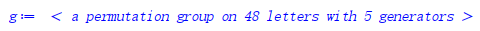But I want to get the `PermutationGroup` form like `PermutationGroup({[[...]], [[...], [...]]})`. Can we change it into this form?

## Is a bug of IsSubgroup?...

As we know, since the order about the element `Perm([[1, 2], [3, 4, 5]])` of S5 is 6. Then  the C6 is a subgroup of S5, but why `IsSubgroup(CyclicGroup(6), SymmetricGroup(5))` return `false`? Is it a bug?

## How to generate a polynomial corresponding to a so...

Of course, we know the A5 of 60 order is an unsolvable group, but as the wiki here, There are also some solvable groups in the same 60 order. Similarly, although `map(IsSolubleNumber, [60, 120, 168, 180])` will give `false`, there are some solvable groups in orders 60, 120, order 168, and order 180. But how to find these corresponding solvable polynomials by maple? I tried to generate them using random polynomials like this:

```with(GroupTheory);
do
do poly := randpoly(x, degree = rand(6 .. 8)()); until irreduc(poly);
G := GaloisGroup(poly, x);
until IsSoluble(G) and is(GroupOrder(G) in {60, 120, 168, 180});
poly;
galois(poly, x);```

But I didn't get any result even after one night..

## Maple 2021 slow...

Anyone experience a delay in typing as the screen fills with text/math etc.?

I'm using Maple 2021 and as the space is filled typing slows. I can finish typing and watch the last 4 keys enter on the screen.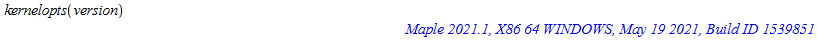## Problem running xmaple 2021.2 in Ubuntu 20.04...

Since approximately a week ago, probably after some Ubuntu update, the xmaple 2021.2 interface started to crash after some simple commands, without any error message or log. I tried to run with java (open-jdk) 11 and 8, and I had the same problem. I still have an old 2017.3 version, and it works perfectly. The problem only affects the new Maple release.

Is there some bug report? What can I do?

## How to get a group that meeting the criteria?...

I'm a newbie to maple, I want to get a group that meeting the criteria with this code:

```with(GroupTheory);
G := SymmetricGroup(6);

do

H := Subgroup({RandomElement(G),RandomElement(G)}, G);

C := Core(H, G);

until is(GroupOrder(C) <> 1) and is(GroupOrder(C) <> GroupOrder(H));
H;
C;```

But it's seem I cannot construct subgroup. so I adjust it into:

```with(GroupTheory);
G := SymmetricGroup(6);

do

H := Subgroup({[[rand(1 .. 6)]], [[rand(1 .. 6), rand(1 .. 6)], [rand(1 .. 6), rand(1 .. 6)]]}, G);

C := Core(H, G);

until is(GroupOrder(C) <> 1) and is(GroupOrder(C) <> GroupOrder(H));
H;
C;```

However, it is clear that random numbers do not always satisfy the rules of permutation. So how can I use the loop to generate a random subgroup that satisfies the condition?

## Count Fridays 13 of a year...

How to adapt this program C to Maple ? Thank you.

Sub Les_Vendredi_13()
Dim Annee As Integer
Dim Mois As Byte
Dim Cellule As String
Dim Compteur As Byte
Cellule = "B2"
Compteur = 1
Columns("B:B")
.ClearContents For Annee = 1949 To 2009
For Mois = 1 To 12 If Weekday(Mois & "/13/" & Annee) = 6
Then Range(Cellule).Offset(Compteur, 0) = "13-" & Format(Mois, "00") & "-" & Annee Compteur = Compteur + 1 End
If Next Mois Next Annee Range(Cellule).Offset(Compteur, 0) = "Nbre = " & Compteur - 1
End Sub

## Sort non-commutative variables with(Physics)...

Hello!

I'd like to sort variables which are non-commutative and obey certain commuting rules in a preferred order. Here is a minimal example

`with(Physics)`

`Setup(mathematicalnotation = true):Setup(noncommutativeprefix = {q, w}, algebrarule = {%Commutator(q, w) = A}) `

If I want to rewrite `q*w` as `A+w*q` since I prefer the order w>q, what should I do? I tried `sort(Simplify(q*w, algebrarules), [w, q]) `but it doesn't work.

## Long form ScientificConstants GetValue not working...

The long form of ScientificConstants[GetValue] is not working in at least Maple 2021 and Maple 2022

ScientificConstants[GetValue](Constant(g))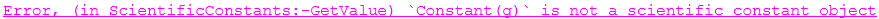This is causing issues for MapleFlow, not being able to call on a constant.

**edit some of the long forms work - specifically the GetValue does not.  I modified the title to reflect that.**

## How do I know exactly which group the GaloisGroup ...

```with(GroupTheory);
G := GaloisGroup(x^5 + 20*x + 32, x);```

I just can get a result like:

`GroupTheory:-GaloisGroup(x^5 + 20*x + 32, x)`

But how do I know exactly what group it is? Actually I know the galois group is D5, But can we use maple to get this information?

## How to get the group in result of DerivedSeries?...

`ds := DerivedSeries(PermutationGroup({[[2, 3, 5, 4]], [[1, 2, 3, 4, 5]]}))`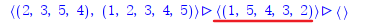How to get the group by the red line point out? Then I can use `GroupOrder` to get the order of it. I note `GroupOrder[ds]` don't work